﻿ Python: Access the index of a list - w3resource# Python: Access the index of a list

## Python List: Exercise - 20 with Solution

Write a Python program access the index of a list.

Example - 1 :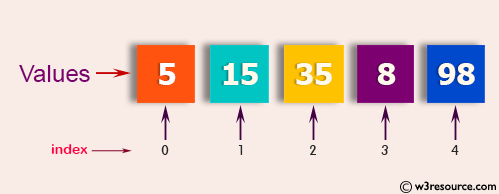Example - 2 :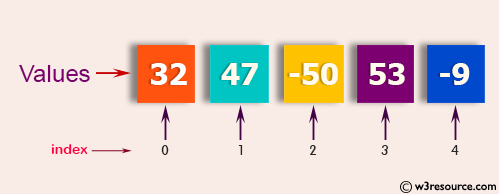Example - 3 :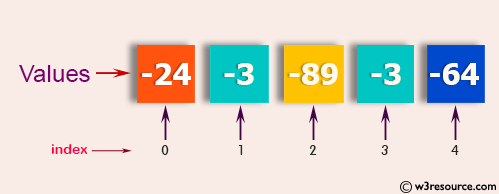Example - 4 :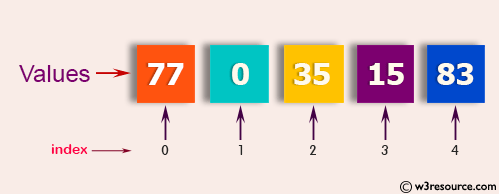Sample Solution:-

Python Code:

``````nums = [5, 15, 35, 8, 98]
for num_index, num_val in enumerate(nums):
print(num_index, num_val)
```
```

Sample Output:

```0 5
1 15
2 35
3 8
4 98
```

Flowchart: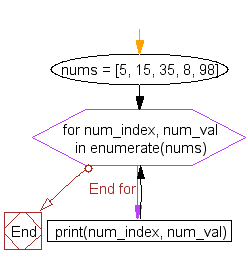## Visualize Python code execution:

The following tool visualize what the computer is doing step-by-step as it executes the said program:

Python Code Editor:

Have another way to solve this solution? Contribute your code (and comments) through Disqus.

What is the difficulty level of this exercise?

Test your Python skills with w3resource's quiz

﻿

## Python: Tips of the Day

Floor Division:

When we speak of division we normally mean (/) float division operator, this will give a precise result in float format with decimals.

For a rounded integer result there is (//) floor division operator in Python. Floor division will only give integer results that are round numbers.

```print(1000 // 300)
print(1000 / 300)```

Output:

```3
3.3333333333333335```